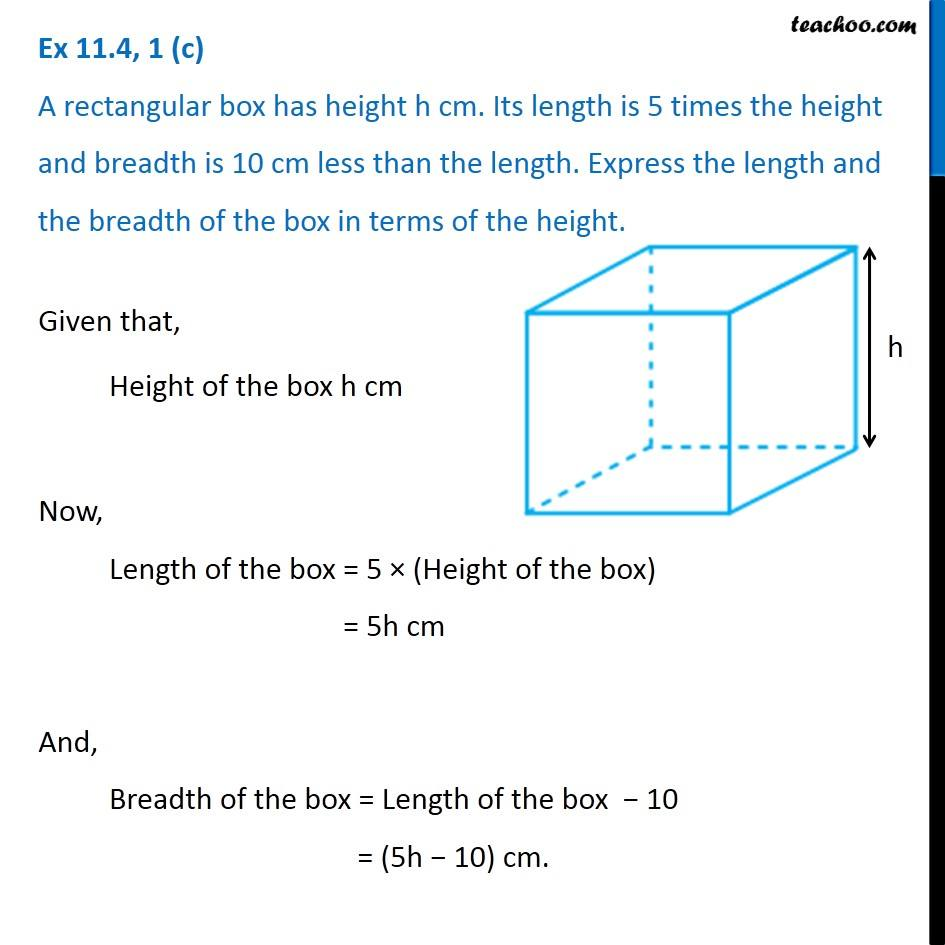Creating Algebra Expressions from Word Problems - Worksheet

Chapter 11 Class 6 Algebra
Serial order wiseLearn in your speed, with individual attention - Teachoo Maths 1-on-1 Class

### Transcript

Question 1 (c) A rectangular box has height h cm. Its length is 5 times the height and breadth is 10 cm less than the length. Express the length and the breadth of the box in terms of the height. Given that, Height of the box h cm Now, Length of the box = 5 × (Height of the box) = 5h cm And, Breadth of the box = Length of the box − 10 = (5h − 10) cm.